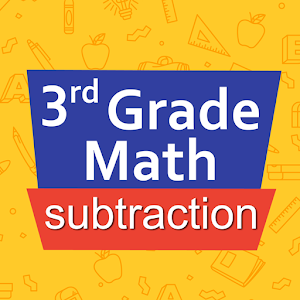Español

Todos los días ofrecemos apliaciones y juegos licenciados GRATIS para Android de otra manera tendrás que comprarlo.2.99
gratis hoy

# Android Giveaway of the Day - Third grade Math - Subtraction

An assistant and training suite for subtraction.
2.99 gratis hoy
Votación de Usuario: 2

está disponible como oferta en Giveaway of the Day!
Tienes tiempo limitado para descargar e instalar.Intuitive interface powered by handwriting input and a fun and engaging mini game in addition to a regular math trainer mode make our app stand out from the crowd of generic math learning apps.

With Third grade Math - Subtraction you can practice and improve the following math skills:
- Subtraction facts - numbers up to 100
- Subtract two two-digit numbers
- Subtract two three-digit numbers
- Complete the subtraction sentence up to three digits
- Subtract numbers with four or five digits
- Balance subtraction equations up to two digits
- Balance subtraction equations up to three digits

Sergey Malugin

Education

### Versión:

Varies with device

### Tamaño:

Varies with device

Everyone